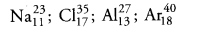# Structure of the Atom Class 9 Important Questions Science Chapter 4

Chapter 4 Structure of the Atom Class 9 Science Important Questions with Answers PDF will help you in scoring more marks in your exams.

## Structure of the Atom Class 9 Important Questions Science and Answers Chapter 4

Question 1.
J. Chadwick discovered a sub-atomic particle which has no charge but has mass nearly equal to that of a proton. Name the particle and give its location in an atom. (CBSE 2011)
The information suggests that the particle discovered by Chadwick is neutron. It is denoted by the symbol 10n. It is located in the nucleus of an atom along with proton.

More Resources

Question 2.
If ‘K’ and ‘L’ shells of an atom are completely filled, then what would be

1. the total number of electrons in an atom and
2. its valency ? (CBSE 2011)

According to Bohr Bury scheme, ‘K’ and ’L’ shells of an atom can have maximum of 2 and 8 electrons respectively. If these are completely filled, this means that :

1. Total number of electrons in the atom = 2 + 8 = 10
2. Valency of the atom = zero.

Question 3.
For chlorine, Z = 17, A = 35. Give the number of protons, electrons and neutrons in

1. chlorine atom
2. chloride ion. (CBSE 2011)

1. In chlorine atom (Cl)
Number of protons (Z) = 17
Number of neutrons = A- Z = 35-17= 18
2. In chloride ion (CF)
Number of protons (Z = 17)
Number of electrons = (Z+1)=17+1 = 18
Number of neutrons = A – Z = 35 – 17 = 18

Question 4.
List three observations of the experiment performed by Rutherford for his model of an atom.
From the scattering experiment, Rutherford made the following observations :

1. Most of the alpha particles were able to pass through the gold foil undeflected.
2. Some of these particles were deflected by small angles.
3. A very few (one out of approximately 12000) alpha particles suffered major deflections and even came back in the same direction.

Question 5.
An atom of an element has two electrons in outermost M-shell. State its
(a) Electronic configuration
(b) Number of protons
(c) Atomic number
(d) Nature whether metal or non-metal
(e) Valency
(f) Name (CBSE 2011)
(a) Since the atom has two electrons in outermost M-shell, this means that K and F shells are already filled. Therefore, electronic configuration is 2(K) 8(F) 2(M)
(b) Number of protons = Number of electrons = 12
(c) Atomic number = Number of protons =12
(d) The elements with two valence electrons is a metal
(e) Valency of the element = Number of outermost electrons = 2
(f) The element is magnesium (Mg)
Hydrogen has three isotopes which are written as :

Question 6.
Explain why :
(a) These isotopes have identical chemical properties.
(b) These isotopes are electrically neutral
(c) These isotopes differ in their masses. (CBSE 2011, 2016)
(a) The isotopes have identical chemical properties because all of them have one electron in the only shell (K-shell)
(b) The isotopes are electrically neutral because each one has one proton and one electron.
(c) The isotopes differ in their masses because they differ in their mass numbers (1,2 and 3 respectively).

Question 7.
In the following table, the mass number and the atomic number of certain elements are given :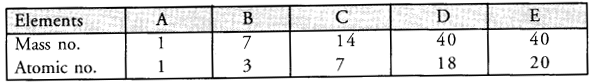(a) Select the pair of isobars from the above table.
(b) What would be the valency of the element C listed in the above table ?
(c) Which two sub-atomic particles are equal in number in a neutral atom ? (CBSE 2011)
(a) Elements D and E are pair of isobars since they have same mass no. = 40
(b) The electronic configuration of the element C with Z = 7 is 2, 5.
It has five valence electrons. Its valency can be either 5 or 3 (8 – 5) = 3.
(c) In a neutral atom, the number of electrons in the extra-nuclear portion is equal to the number of protons in the nucleus.

Question 8.
(a) Why are the chemical properties of the isotopes same ?
(b) Draw Bohr model for helium atom.
(c) What are the number of protons, neutrons and electrons in 5927Co and 10847Ag ? (CBSE 2011)
(a) Isotopes have the same atomic number as well as the same electronic configuration. Therefore, their chemical properties are the same.
(b)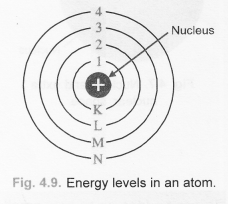(c)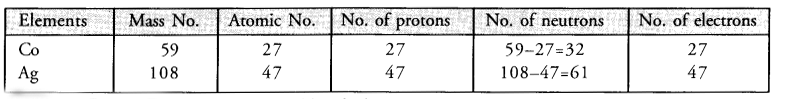Question 9.
(a) Which popular experiment is shown in the figure ?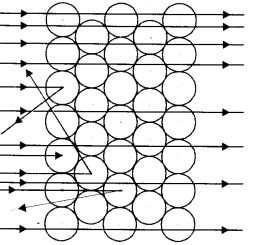(b) List three observations of this experiment.
(c) State conclusions drawn from each observation of this experiment.
(d) State the model of atom suggested on the basis of the above experiment. (CBSE 2011)
(a) Rutherford  Model
(b)  Rutherford made the following observations :

1. Most of the alpha particles were able to pass through the gold foil undeflected.
2. Some of these particles were deflected by small angles.
3. A very few (one out of approximately 12000) alpha particles suffered major deflections and even came back in the same direction.

(c) Conclusions

1. As most of the alpha particles passed through undeflected, this means that they did not come across any obstruction in their path. Thus, most of the space in an atom is expected to be empty.
2. As a few alpha particles suffered minor deflections and a very few major deflections, this means that these must have met with some obstructions in their path.
3. This obstruction must be :
1. Very small : Only a few particles were obstructed by it.
2. Massive : Each alpha particle has 4u mass and is quite heavy. It could easily pass through a light obstruction by pushing it aside.

(d) The main features are listed as follows :

1. An atom consists of two parts. These are nucleus and extra nuclear portion.
2. Nucleus is present in the centre of the atom and is surrounded by extra nuclear portion.
3. The radius of the nucleus of an atom is nearly 10-15m while that of the atom is about 10-10m. Thus, nucleus is very small in comparison to the atom.
4. The mass of the atom is mainly of the nucleus. All the protons and neutrons (discovered later on by Chadwick) are present in the nucleus.
5. The positive charge on the nucleus is because of protons present (each proton has one unit positive charge).
6. All the electrons are present in the extra-nuclear space around the nucleus.
7. The total positive charge of the nucleus due to the presence of protons is the same as that of the electrons present in the extra nuclear space. Therefore, the atom as a whole is electrically neutral.
8. Electrons present in the extra nuclear portion are not stationary. These are revolving around the nucleus at high speed following a circular path.
9. The revolving electrons do not come close to the nucleus or drawn towards the nucleus because their force of attraction towards the nucleus is balanced by the centrifugal force which is of the same magnitude. It is directed away from the nucleus

Question 10.
There are two elements 2613A and 2614B. Find the number of sub-atomic particles in each of these. What is the relation between these atoms ?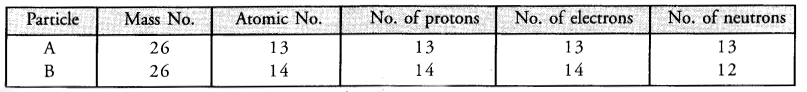Since the two elements have same mass number hut different atomic numbers, these are related to each other as isobars.

Question 11.
(a) In the gold foil experiment, what observations led Rutherford to conclude that
(i) Most of the space inside the atom is hollow.
(ii) The central portion of the atom is positively charged.
(iii) Volume occupied by the nucleus is very small as compared to the total volume of the atom.
(iv) Almost the entire mass of the atom concentrated at its centre.
(b) If bromine atom is available in the form of two isotopes 7935Br (49.7%) and 8135Br (50.3%), calculate the average atomic mass of bromine atom. (CBSE 2014, 2016)
(a) (i) Most of the α-particles passed through the foil undeflected.
(ii) Some α-particles (positively charged) were deflected and a few came back in the same direction. This means that there is very small, positively charged and massive portion present in the centre of the atom. This is known as nucleus.
(iii) The number of α-particles which were deflected back was very small.
(iv) Since the mass of the atom is mainly due to positively charged protons (neutrons were not known at that time), this means that the entire mass of the atom is concentrated in the centre of the atom called nucleus.
(b) % of Br isotope with mass number 79 = 49.7 % of Br isotope with mass number 81 = 50.3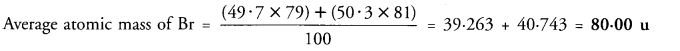Question 12.
State the postulates stated by Neils Bohr in order to overcome the objection as raised against Rutherford’s model of atom.
The main postulates of the theory are listed :

1. In the extra nuclear portion of an atom, the electrons revolve in well defined circular paths known as orbits.
2. These circular orbits are also known as energy levels or energy shells.
3. These have been designated as K, L, M, N, O, … (or as 1, 2, 3, 4, 5, …) based on the energy present.
4. The order of the energy of these energy shells is :
K<L<M<N<0 <…. or 1< 2< 3 < 4<5 <….
5. While revolving in an orbit, the electron is not in a position to either lose or gain energy. In other words, its energy remains stationary. Therefore, these energy states for the electrons are also known as stationary states.

Question 13.
Which of the following are isotopes and which are isobars ?
Argon, Protium, Calcium, Deuterium. Explain why the isotopes have similar chemical properties but they differ in physical properties ? (CBSE 2012)
The symbol notations for elements are represented as follows :
11H and 21H are isotopes while 4018Ar and 4020Ca are isobars. Since the isotopes of an element have same atomic number, they have same electronic configuration and also similar chemical properties. They have different physical properties since their mass numbers are different.

Question 14.
Explain Bohr and Bury rules for distribution of electrons into different shells.

1. The maximum number of electrons which can be present in a particular energy shell of an atom is given by 2n2. Here ‘n is the number of the energy shells or energy levels.
2. The outermost energy shell in an atom cannot have more than eight electrons even if it has a capacity to take up more electrons according to first rule.
3. It is not necessary for a given shell to complete itself before another shell starts forming. As a rule, the new shell is formed as soon as the outermost shell, acquires eight electrons.

Question 15.
The atomic number and mass number of an element are 16 and 32 respectively. Find the number of protons, electrons and neutrons in it. State its valency. Is this element a metal or a non – metal. Justify your, answer.
(CBSE 2012, 2014, 2016)
No. of protons = Atomic number =16
No. of electrons = Atomic number =16
No. of neutrons = Mass number – Atomic number = 32-16 = 16
Electronic configuration of the element = 2, 8, 6
Valency of the element = (8 – 6) = 2
The element is a non – metal since it tends to gain electrons and not lose electrons.

Question 16.
The K and L shells of an atom are completely filled. Find the number of electrons present in it. State the name of the element. . (CBSE 2012, 2013)
Number of electrons present : K(2), L(8) = 10. The element is neon (Ne).

Question 17.
You are given an element X. Find out
(a) Number of protons, electrons and neutrons in ‘X’.
(b) Valency of ‘X’ ,
(c) Write the chemical formula of the compound formed when ‘X’ reacts with
(i) hydrogen,
(ii) carbon.
(CBSE 2012)
(a) Number of protons = 8
Number of electrons = 8 Number of neutrons = 16 – 8 = 8
Electronic configuration = 2, 6
(b) Valency of ‘X’ =8-6 = 2
(c) The element ‘X’ is ‘O’. The formula of the compounds with hydrogen (H) and oxygen (O) are :
(i) H2O (ii) CO2.

Question 18.
Define the terms (a) isotope, (b) isobar giving one example in each case. Name the element whose isotope is used in
(i) nuclear reactor,
(ii) treatment of cancer. (CBSE 2012)
(a) Most of the elements exist in nature. An investigation of some of these have revealed that the atoms of a particular element may have different mass numbers. However, their atomic numbers are the same. These are called isotopes. Thus, isotopes may be defined as : the different atoms of the same element having same atomic number but different mass numbers.
(b)  Isobars may be defined as : the atoms belonging to the different elements with same mass numbers but different atomic numbers.
(i) U-235 isotope is used in nuclear reactor as a fuel.
(ii) Co-60 isotope is used in the treatment of cancer.

Question 19.
(a) What are canal rays ? Who discovered them ? What is the charge and mass of canal rays ?
(b) How are canal rays different from electrons in terms of charge and mass ? (CBSE 2012)
(a) Canal rays are the rays which originate in the discharge tube experiment and move away from the anode. These are called canal rays since they seem to be flowing like a stream of water in a canal.
(b) Canal rays are basically anode rays which consist of protons. For the charge and mass of protons. For the comparison of canal rays and electrons in terms of charge and mass.

Question 20.
A certain particle X has 17 protons, 17 electrons and 18 neutrons
(i) What is the mass number of X ?
(ii) What is atomic number of X ?
(iii) What is valency of X ?
(iv) Identify the element.
(i) Mass no. of X = No. of p + No. of n = 17 + 18 = 35
(ii) Atomic no. of X = No. of p = 17
(iii) Electronic configuration of X = 2, 8, 7
Valency of X = (8 – 7) = 1
(iv) Name of element X = Chlorine (Cl).

Question 21.
Who discovered protons ?
Protons were discovered by Goldstein.

Question 22.
(a) The element helium has 2 electrons in its valence shell but its valency is not 2. Explain.
(b) Choose the isotopes from the following nuclei :
(i) 8p + 8n
(it) 8p + 9n
(iii) 18p + 22n
(iv) 20p + 20n (CBSE 2013)
(a) The element helium (He) is a noble gas element (Z = 2). It has only one shell (K shell) which can have maximum of two electrons only. It therefore, does not take part in chemical combination and its valency is zero.
(b) Elements (i) and (ii) represent pair of isotopes since they have 8 protons (Atomic no. = 8)

Question 23.
What are valence shell and valence electrons ? (CBSE 2013)
Valence shell is the outermost shell in an atom. The electrons present in it are called valence electrons.

Question 24.
Complete the following table :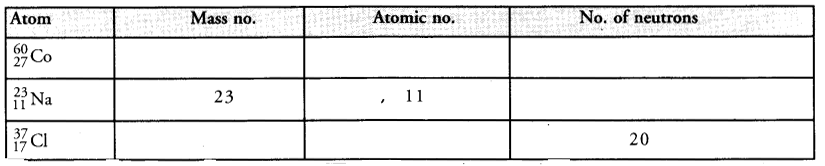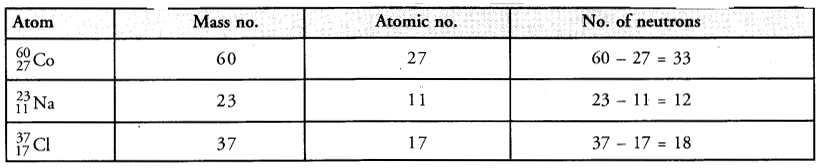Question 25.
The total number of nucleons in the atoms of calcium and argon is 40 and the atomic numbers of calcium and argon are 20 and 18 respectively. Name the pair of these two elements and also find out the number of neutrons present in the nucleus of argon atom. (CBSE 2013)
The elements which have same no. of nucleons but different atomic numbers are called isobars. Therefore, calcium (Ca) and argon (Ar) represent a pair of isobars.
No. of neutrons in the nucleus of Ar = 40 – 18 = 22.

Question 26.
Atom of an element has one proton, one electron and no neutron. Name the element. How will you represent it ? (CBSE 2013)
The element is called protium or hydrogen. It is represented as j H

Question 27.
An atom has 2 electrons in M-shell. What is the atomic number of the element ? (CBSE 2014)
K and L shells of the atom are filled and M shell has two electrons. Therefore,
Total number of electrons in the atom = 2 + 8 + 2= 12
Atomic number (Z) of the element = 12.

Question 28.
An element is represented as 168X. Find :
(a) The number of electrons in element X. .
(b) Mass number of an element X.
(c) The number of neutrons in element X. (CBSE 2014, 2016)
(a) The number of electrons in element X = 8
(b) Mass number of element X = 16
(c) The number of neutrons in element X = 16-8 = 8.

Question 29.
The electronic configuration of potassium (K) is 2,8,8, 1 instead of 2,8,9 though the M shell can accommodate up to 18 electrons. Explain. (CBSE 2014)
M-shell cannot have more than 8 electrons in case it happens to be outermost or valence shell in an atom. Therefore, the correct electronic configuration of the element potassium (K) is 2,8,8,1.

Question 30.
Show the electron distribution in magnesium atom and magnesium ion diagrammatically and also give their atomic numbers. (CBSE 2014)
Atomic number (Z) of magnesium is 12. Its electronic distribution is 2,8,2. Magnesium ion (Mg2+) is formed by the loss of two electrons from the valence shell of the atom. Therefore, the electronic distribution * in the ion is 2, 8. These are shown diagrammatically as follows :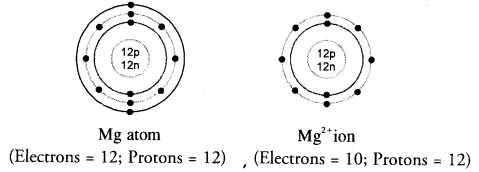Question 31.
The composition of two atoms A and B is given :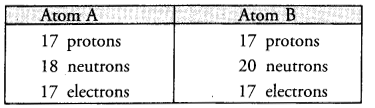(a) What are the mass numbers and atomic numbers of A and B ?
(b) What is the relation between the two chemical species ?
(c) Which element or elements do they represent ?
(a) Mass number of A = 17 + 18 = 35 u
Mass number of B = 17 + 20 = 37 u
(b) The two chemical species exist as pair of isotopes since they have the same number of protons and electrons.
(c) They represent the element chlorine (Cl).

Question 32.
Write the names of three elementary particles which constitute an atom. (CBSE 2015)
Three elementary particles which constitute an atom are ; electron (e), proton (p) and neutron (n).

Question 33.
(a) What is the relationship between two elements X and Y whose atomic numbers are 18 and 20 respectively but their mass numbers remain the same as 40 ?
(b) Are their chemical properties same or different ? Explain and support your answer.
(c) Which has more number of electrons Na or Na+ ? Why ? (CBSE 2015)
(a) The elements X and Y are related to each other as isobars.
(b) Since the atomic numbers of the elements are different, their chemical properties also differ.
(c) The electronic configurations of Na and Na+ ion are given as follows :
Na (Z =11), K(2), L(8), M(l)
Na+ (Z = 11), K(2), L(8), M(-)
Na+ ion is formed when Na atom loses one electron. Therefore, Na atom has more electrons (11) than Na+ ion (10).

Question 34.
Write the electron distribution of oxygen atom. How many valence electrons does it have ? (CBSE 2015)
Atomic number (Z) of oxygen is 8.
Electronic distribution = K(L), L(6)
Valence electrons = 6.

Question 35.
(a) Why does helium have zero valency ?
(b) Name the scientist and his experiment to prove that nucleus of an atom is positively charged.
(CBSE 2015)
(a) Atomic number (Z) of helium is 2. Its electronic configuration is K(2). This means that its atom has completely filled shell which is the only shell it has. Therefore, valency of the element is zero.
(b) The scientist is Rutherford and the experiment is known as α-particle scattering experiment.

Question 36.
List the observations in α-particle scattering experiment which led Rutherford to make the following conclusions :
(i) Most of the space in an atom is empty,
(ii) Whole mass of an atom is concentrated in its centre.
(iii) Centre is positively charged. (CBSE 2015)
(i) As most of the alpha particles passed through undeflected, this means that they did not come across any obstruction in their path. Thus, most of the space in an atom is expected to be empty.
(ii)  As a few alpha particles suffered minor deflections and a very few major deflections, this means that these must have met with some obstructions in their path.
This obstruction must be :

• Very small : Only a few particles were obstructed by it.
• Massive : Each alpha particle has 4u mass and is quite heavy. It could easily pass through a light obstruction by pushing it aside.

(iii) Positively charged : Alpha particles have positive charge. Since they were repelled or deflected back, the obstruction must also carry same charge i.e., positive charge, (similarly charged particles always repel each other).

Question 37.
Define isotopes. Why do isotopes have same atomic number but different mass numbers ? Explain with the help of an example. (CBSE 2015)
Most of the elements exist in nature. An investigation of some of these have revealed that the atoms of a particular element may have different mass numbers. However, their atomic numbers are the same. These are called isotopes. Thus, isotopes may be defined as :
The different atoms of the same element having same atomic number but different mass numbers.
Please note that the difference in the mass numbers of the isotopes is because of the difference in the number of neutrons present. For example,
Hydrogen (H) exists in the form of three isotopes. These are named as :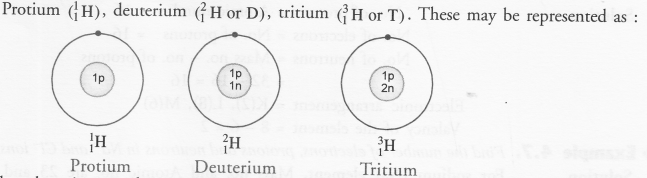Question 38.
Write the two postulates of Thomson’s model of an atom. What were the drawbacks in this model ?
(CBSE 2015)

1. An atom may be regarded as a positively charged sphere in which protons are present.
2. The negatively charged electrons may be regarded as studded or embedded in this sphere.

Question 39.
(a) How many neutrons are present in C-14 isotope of carbon ?
(b) How many protons does He2+ ion possess ?
(c) How many electrons can be filled in the third orbit of an atom at the maximum ? (CBSE 2015)
(a) C-14 isotope of carbon has mass number 14 and atomic number 6. In this isotope ;
No. of protons = Atomic no. = 6.
No. of electrons = Atomic no. = 6.
No. of neutrons = Mass no. – Atomic no.
= 14 – 6 = 8.
(b) He2+ ion (z = 2) has two protons,
(c) The third orbit of an atom can have maximum of 8 electrons.

Question 40.
Identify the most stable atom from the following. Also give the reason for your answer :